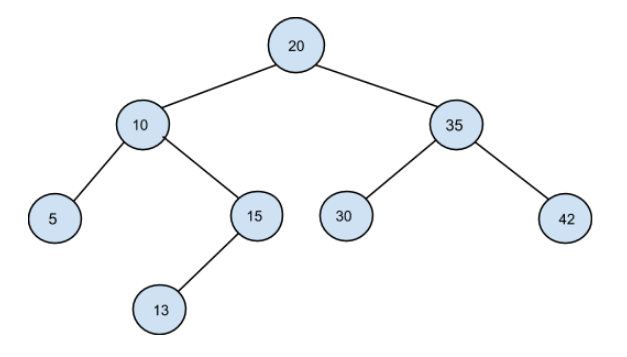# Largest Common Ancestor

Posted: 9 Oct, 2020
Difficulty: Moderate

## PROBLEM STATEMENT

#### Largest Common Ancestor is the one having the highest node value among all the common ancestors of given nodes. Assume both nodes are present in the binary tree.

##### Input Format:
``````The first line of input contains an integer T, the number of test cases.

The first line of every next T lines contain elements in the level order form. The input consists of values of nodes separated by a single space in a single line. In case a node is null, we take -1 on its place.

The second line of every next T lines contains Node 1’s data.

The third line of every next T lines contains Node 2’s data.
``````
##### Note
``````For example, the input for the tree depicted in the below image would be :
````````````20
10 35
5 15 30 42
-1 13 -1 -1 -1 -1 -1
-1 -1

Explanation:

Level 1 :
The root node of the tree is 20
Level 2 :
Left child of 20 = 10
Right child of 20 = 35
Level 3 :
Left child of 10 = 5
Right child of 10 = 15
Left child of 35 = 30
Right child of 35 = 42
Level 4 :
Left child of 5 = null (-1)
Right child of 5 = null (-1)
Left child of 15 = 13
Right child of 15 = null (-1)
Left child of 30 = null (-1)
Right child of 30 = null (-1)
Left child of 42 = null (-1)
Right child of 42 = null (-1)
Level 5 :
Left child of 13 = null (-1)
Right child of 13 = null (-1)
The first not-null node (of the previous level) is treated as the parent of the first two nodes of the current level. The second not-null node (of the previous level) is treated as the parent node for the next two nodes of the current level and so on.
The input ends when all nodes at the last level are null (-1).

Note:
The above format was just to provide clarity on how the input is formed for a given tree.
The sequence will be put together in a single line separated by a single space. Hence, for the above-depicted tree, the input will be given as:
20 10 35 5 15 30 42 -1 13 -1 -1 -1 -1 -1 -1 -1
``````
##### Output Format:
``````For every test case print single line containing an integer i.e the largest common ancestor.
``````
##### Note:
``````You do not need to print anything, it has already been taken care of. Just implement the given function.
``````
##### Constraints:
``````1 <= T <= 5
1 <= N <= 10^5
0 <= data <= 10^9

Time limit: 1 second
``````Approach 1
• Maintain an array, say Path1 and store the path from the root to Node1 in that.
For example, for the input:
Level order traversal: 8 5 10 2 6 -1 -1 -1 -1 -1 7 -1 -1
Node 1: 2
Node 2: 7
Path 1= { 8, 5, 2 }
• Maintain another array, say Path2, and store the path from the root to Node2 in that.
Thus, Path 2= { 8, 5, 6, 7 }
• Traverse the 2 arrays Path1 and Path2 from start i.e. Path1 to the point where the value of Path1[i] is not equal to Path2[i].
When the value of Path1[i] and Path2[i] are not equal, it means that the paths to node1 and node2 diverged at index ‘i-1’ and there is no common ancestor after index i.
As in the above example, traverse till index 1 for a 0 indexed array.
• Maintain the maximum value as that will be the answer. In this case, 8.

Algorithm: to find a path from the root to node x

list<int> getPath(root, x)

• Initialize a vector.
• While root’s data is not equal to x
• Push_back root’s data.
• If root’s value is greater than x, update root to root’s left.
• Otherwise, update root to root’s right.
• Push_back root’s data.

Algorithm: to find LCA of two nodes data1 and data2

Int LargestCommonAncestor(root, data1, data2)

• vector<int> A = getPath(root, data1)
• vector<int> B = getPath(root, data2)
• Initialize a variable to store max value.
• Initialise index variable with 0.
• While the index is less than the size of A as well as B
• If A[index] != B[index], break.
• If A[index] > maximum, maximum = A[index]
• Increment index by 1
• Return Maximum.